L's in Circles

The following pictures show n L's with short side 1 packed inside the smallest circle (of radius r).

 1.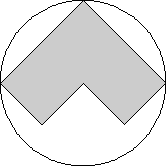2.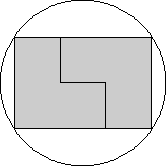3.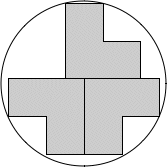r = √2 = 1.414+Trivial. r = √13/2 = 1.802+Found by David W. Cantrellin June 2005. r = √305/8 = 2.183+Found by Maurizio Morandiin August 2007.

 4.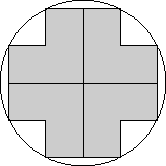5.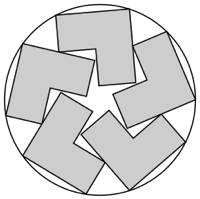6.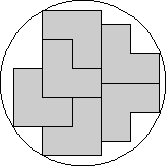r = √5 = 2.236+Found by Erich Friedmanin June 2005. r = 2.569+Found by Maxim Razin and Nick Baxterin November 2019. r = √8249/32 = 2.838+Found by Erich Friedmanin June 2005.

 7.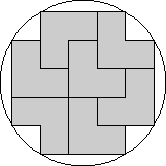8.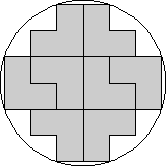9.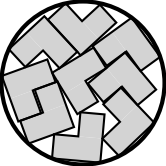r = √34/2 = 2.915+Found by Erich Friedmanin June 2005. r = √10 = 3.162+Found by Erich Friedmanin June 2005. r = 3.373+Found by David W. Cantrellin August 2007.

 10.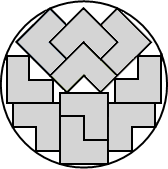11.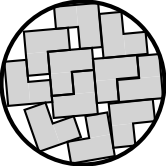12.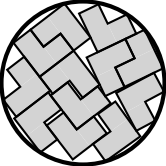r = 3.516+Found by Maurizio Morandiin August 2007. r = 3.705+Found by Maurizio Morandiin September 2008. r = √58/2 = 3.807+Found by Erich Friedmanin June 2005.

 13.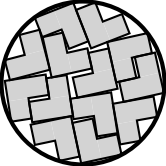14.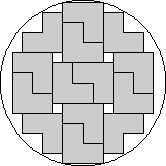15.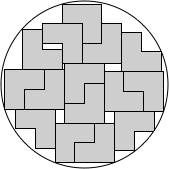r = 3.958+Found by Maurizio Morandiin September 2008. r = √17 = 4.123+Found by David W. Cantrellin August 2007. r = 4.214+Found by Maurizio Morandiin August 2007.

 16.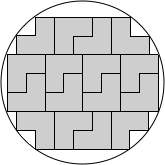17.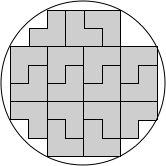18.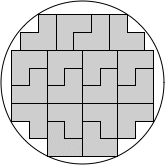r = √74/2 = 4.301+Found by Maurizio Morandiin September 2008. r = 2√5 = 4.472+Found by Maurizio Morandiin September 2008. r = √86609/64 = 4.598+Found by Maurizio Morandiin September 2008.